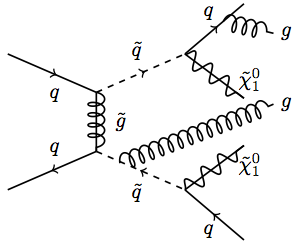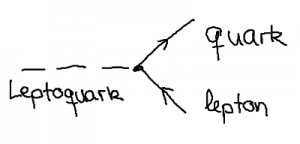Categories
Pages
-Super-precision for the Large Hadron Collider

February 5th, 2015 | by

PhD student Mathieu Pellen describes his research on precision calculations for supersymmetry as published in two recent scientific articles (open access versions can be found here and here).

Despite the discovery of the Higgs boson, numerous theoretical issues in particles physics remain unexplained. This is the reason why new theories are required. These theories can be tested in experiments such as the LHC (Large Hadron Collider, CERN, Geneva) and supersymmetry is one of the best motivated theories beyond the standard model. It is thus a major task of the experimental collaborations to search for supersymmetric particles. So far no sign of the existence of supersymmetry in collider experiments has been seen. Nonetheless, there is still lots of room for supersymmetry to be discovered and the next run of the LHC might unravel its nature.

In order to match the unprecedented accuracy of experimental measurements, precise and appropriate theoretical predictions are required. This is achieved by calculating supersymmetric processes with high accuracy. This means calculating it at next-to-leading order (NLO), i.e. the second order in perturbation theory. In addition to this, in order to have more realistic predictions, these calculations have to be matched with so-called parton showers that account for further radiations of quarks and gluons. The aim of this article has thus been to perform a calculation of squark-antisquark (superpartners of the quark) production supplemented by their decay at NLO in perturbation theory and matched with parton showers. The conclusion of this study is that precise predictions in supersymmetric theories are important for LHC phenomenology.Exemplary diagram of NLO calculation matched with parton shower in supersymmetry. The particles with a tilde are supersymmetric particles.

Supersymmetry or just a bunch of logarithms?

November 20th, 2014 | by

In its first phase from 2010 to 2013, CERN’s Large Hadron Collider has delivered an impressive amount of new results. The LHC experiments ATLAS and CMS have measured a myriad of particle scattering cross sections with unprecedented accuracy. These cross sections reflect the probability of producing certain particles in the collisions of protons, smashed at each other by the LHC. The so-called “Stairway to Heaven” plot below shows the remarkable agreement between experimental cross section measurements (points) and the theoretical predictions (lines) within the Standard Model of particle physics. Read the rest of this entry »

Are there leptoquarks?

July 16th, 2014 | byLeptoquarks are interesting objects: These (so far) hypothetical particles are able to connect a single Lepton and a single quark in one interaction. For triggering such an interaction, leptoquarks have to carry both lepton number (such as leptons) and baryon quantum number (such as quarks). Hence the name: Lept-o-quark.

A Higgs or not a Higgs?

July 12th, 2014 | byIt has been exactly two years now since the two big experiments Atlas and CMS at the LHC announced the discovery of a new boson. This boson is so far hotly tipped to be the Standard Model Higgs particle. What does “being the Standard model Higgs particle” mean, are particle physicists sure about that and how do they come to their conclusion?